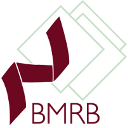Subsections

## bmrb.script### Synopsis

Specify the scripts used in the analysis.

### Defaults

bmrb.script(file=None, dir=None, analysis_type=None, model_selection=None, engine=`relax', model_elim=False, universal_solution=False)

### Keyword arguments

file: The name of the script file.

dir: The directory where the file is located.

analysis_type: The type of analysis performed.

model_selection: The model selection technique used, if relevant. For example `AIC' model selection.

engine: The software engine used in the analysis.

model_elim: A model-free specific flag specifying if model elimination was performed.

universal_solution: A model-free specific flag specifying if the universal solution was sought after.

### Description

This user function allows scripts used in the analysis to be included in the BMRB deposition. The following addition information may need to be specified with the script.

The analysis type must be set. Allowable values include all the data pipe types used in relax, ie:

`frame order' -
The Frame Order theories,
`jw' -
Reduced spectral density mapping,
`mf' -
Model-free analysis,
`N-state' -
N-state model of domain motions,
`noe' -
`relax_fit' -
Relaxation curve fitting,

The model selection technique only needs to be set if the script selects between different mathematical models. This can be anything, but the following are recommended:

`AIC' -
Akaike's Information Criteria.
`AICc' -
Small sample size corrected AIC.
`BIC' -
Bayesian or Schwarz Information Criteria.
`Bootstrap' -
Bootstrap model selection.
`CV' -
Single-item-out cross-validation.
`Expect' -
The expected overall discrepancy (the true values of the parameters are required).
`Farrow' -
Old model-free method by Farrow et al., 1994.
`Palmer' -
Old model-free method by Mandel et al., 1995.
`Overall' -
The realised overall discrepancy (the true values of the parameters are required).

The engine is the software used in the calculation, optimisation, etc. This can be anything, but those recognised by relax (automatic program info, citations, etc. added) include:

`relax' -
hence relax was used for the full analysis.
`modelfree4' -
Art Palmer's Modelfree4 program was used for optimising the model-free parameter values.
`dasha' -
The Dasha program was used for optimising the model-free parameter values.
`curvefit' -
Art Palmer's curvefit program was used to determine the R1 or R2 values.

The model_elim flag is model-free specific and should be set if the methods from "d'Auvergne, E. J. and Gooley, P.. (2006). Model-free model elimination: A new step in the model-free dynamic analysis of NMR relaxation data. J. Biomol. NMR, 35(2), 117-135." were used. This should be set to True for the full_analysis.py script.

The universal_solution flag is model-free specific and should be set if the methods from "d'Auvergne E. J., Gooley P.. (2007). Set theory formulation of the model-free problem and the diffusion seeded model-free paradigm. Mol. Biosyst., 3(7), 483-494." were used. This should be set to True for the full_analysis.py script.

### Prompt examples

For BMRB deposition, to specify that the full_analysis.py script was used, type one of:

```[numbers=none]
relax> bmrb.script('full_analysis.py', 'model-free', 'AIC', 'relax', True, True)
```

```[numbers=none]
relax> bmrb.script(file='full_analysis.py', dir=None, analysis_type='model-free', model_selection='AIC', engine='relax', model_elim=True, universal_solution=True)
```

The relax user manual (PDF), created 2020-08-26.